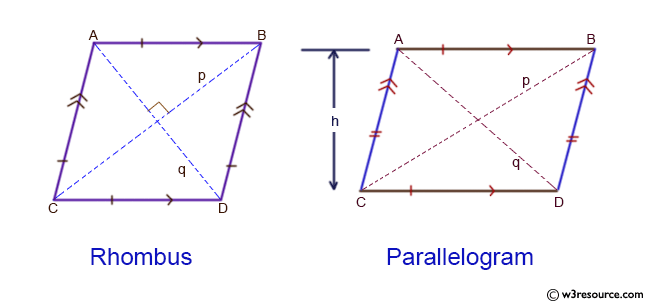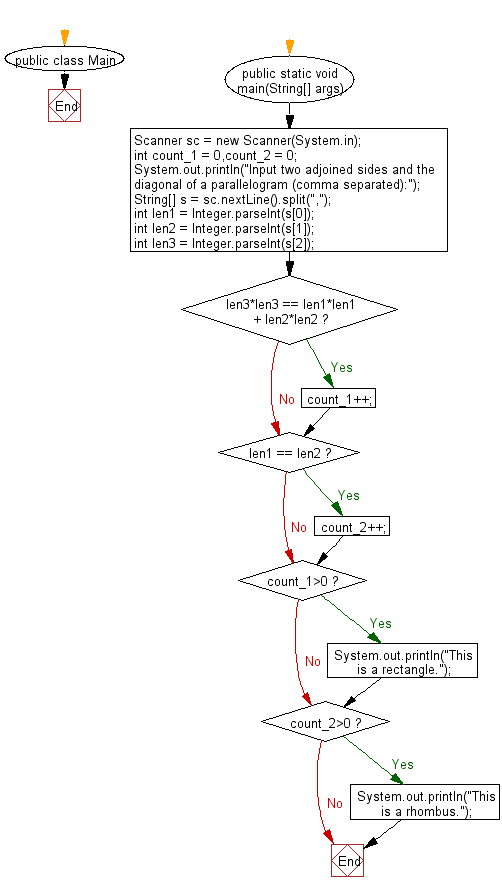# Java Exercises: Reads the two adjoined sides and the diagonal of a parallelogram and check whether the parallelogram is a rectangle or a rhombus

## Java Basic: Exercise-229 with Solution

Write a Java program which reads the two adjoined sides and the diagonal of a parallelogram and check whether the parallelogram is a rectangle or a rhombus.

According to Wikipedia-
parallelograms: In Euclidean geometry, a parallelogram is a simple (non-self-intersecting) quadrilateral with two pairs of parallel sides. The opposite or facing sides of a parallelogram are of equal length and the opposite angles of a parallelogram are of equal measure.
rectangles: In Euclidean plane geometry, a rectangle is a quadrilateral with four right angles. It can also be defined as an equiangular quadrilateral, since equiangular means that all of its angles are equal (360°/4 = 90°). It can also be defined as a parallelogram containing a right angle.
rhombus: In plane Euclidean geometry, a rhombus (plural rhombi or rhombuses) is a simple (non-self-intersecting) quadrilateral whose four sides all have the same length. Another name is equilateral quadrilateral, since equilateral means that all of its sides are equal in length. The rhombus is often called a diamond, after the diamonds suit in playing cards which resembles the projection of an octahedral diamond, or a lozenge, though the former sometimes refers specifically to a rhombus with a 60° angle (see Polyiamond), and the latter sometimes refers specifically to a rhombus with a 45° angle.

Input:

Two adjoined sides and the diagonal.
1 ≤ ai, bi, ci ≤ 1000, ai + bi > ci

Pictorial Presentation:Sample Solution:

Java Code:

`````` import java.util.*;
public class Main {
public static void main(String[] args){
Scanner sc = new Scanner(System.in);
int count_1 = 0,count_2 = 0;
System.out.println("Input two adjoined sides and the diagonal of a parallelogram (comma separated):");
String[] s = sc.nextLine().split(",");
int len1 = Integer.parseInt(s);
int len2 = Integer.parseInt(s);
int len3 = Integer.parseInt(s);
if(len3*len3 == len1*len1 + len2*len2) count_1++;
if(len1 == len2) count_2++;
if(count_1>0)
System.out.println("This is a rectangle.");
if(count_2>0)
System.out.println("This is a rhombus.");
}
}
```
```

Sample Output:

```Input two adjoined sides and the diagonal of a parallelogram (comma separated):
8,8,8
This is a rhombus.
```

Flowchart:Java Code Editor:

What is the difficulty level of this exercise?

﻿

## Java: Tips of the Day

Array vs ArrayLists:

The main difference between these two is that an Array is of fixed size so once you have created an Array you cannot change it but the ArrayList is not of fixed size. You can create instances of ArrayLists without specifying its size. So if you create such instances of an ArrayList without specifying its size Java will create an instance of an ArrayList of default size.

Once an ArrayList is full it re-sizes itself. In fact, an ArrayList is internally supported by an array. So when an ArrayList is resized it will slow down its performance a bit as the contents of the old Array must be copied to a new Array.

At the same time, it's compulsory to specify the size of an Array directly or indirectly while creating it. And also Arrays can store both primitives and objects while ArrayLists only can store objects.

Ref: https://bit.ly/3o8L2KH